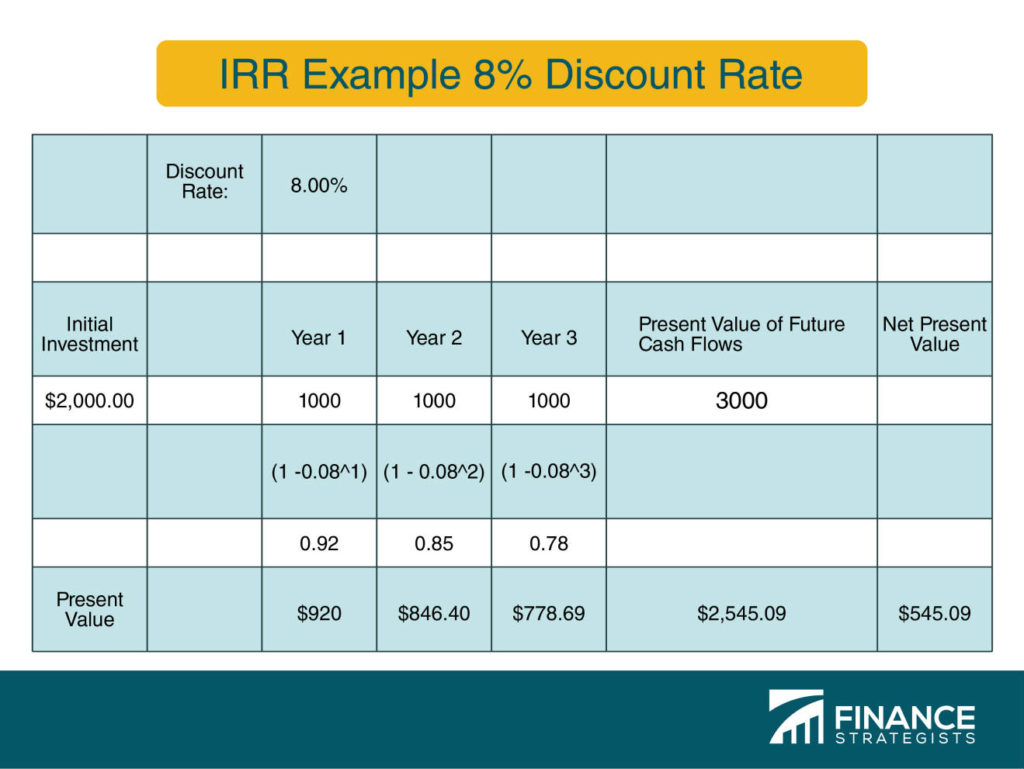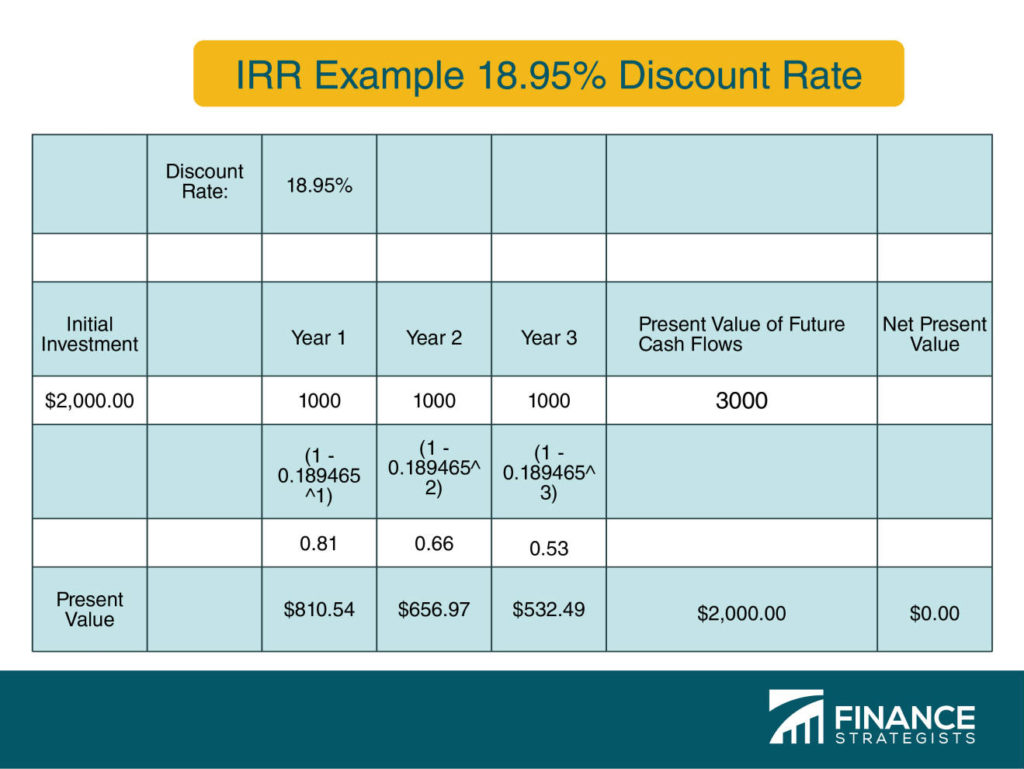# Internal Rate of Return (IRR)### Written byTrue Tamplin, BSc, CEPF® | Reviewed by Editorial Team

Updated on December 14, 2022

## What Is IRR (Internal Rate of Return)?

Internal Rate of Return, or IRR, is the rate of return at which a project breaks even and is used by management to evaluate potential investments.

IRR functions as a return on investment (ROI) calculation.<h2>Foundations of IRR: Net Present Value (NPV)</h2> To understand IRR, one must understand net present value (NPV).

Net present value is calculated by discounting future cash flows by a discount rate, which is the rate a company expects to earn on investments.

## How to Calculate IRR | Example

If a project offers \$1,000 for 3 years, the present value of future cash flows is not worth \$3,000 to the company because it has an expectation that its money should earn money over time.

This concept is known as the “time value of money” (TVM).

## Calculating IRR | Case Study

If a company invests \$2,000 into a project which offers \$1,000 for 3 years at an 8% discount rate, the present value of future cash flows is \$2,545.09.

After subtracting the initial investment, the net present value of the project is \$545.09, suggesting this is a good investment at the current discount rate.The internal rate of return is the discount rate that would bring this project to breakeven, or \$0 NPV.

In this case, an internal rate of return of 18.95% brings the net present value of future cash flows to 0.## IRR Calculation | Hurdle Rate

A company’s discount rate is typically derived from its cost of capital, or the cost a company pays investors in exchange for capital, either in interest from issued debt or through selling equity in the company.

The weighted average cost of capital often functions as a company’s “hurdle rate,” or the minimum required rate of return.

Because the IRR in our example exceeds the discount rate (or required rate of return), the IRR rule says that management should invest in this project.

## IRR Formula Limitations - Opting for the MIRR

It’s important to note that certain assumptions can cause the IRR to become overstated.

For this reason, many investors use the Modified Internal Rate of Return, or MIRR, which account for these assumptions.

## Internal Rate of Return (IRR) FAQs

### What does IRR stand for?

IRR is an acronym for Internal Rate of Return.

### What is an Internal Rate of Return?

Internal Rate of Return, or IRR, is the rate of return at which a project breaks even and is used by management to evaluate potential investments.

### How does an IRR function?

IRR functions as a return on investment (ROI) calculation.

### How is the IRR calculated?

A company’s discount rate is typically derived from its cost of capital, or the cost a company pays investors in exchange for capital, either in interest from issued debt or through selling equity in the company.

### What is the MIRR?

It’s important to note that certain assumptions can cause the IRR to become overstated. For this reason, many investors use the Modified Internal Rate of Return, or MIRR, which accounts for these assumptions.